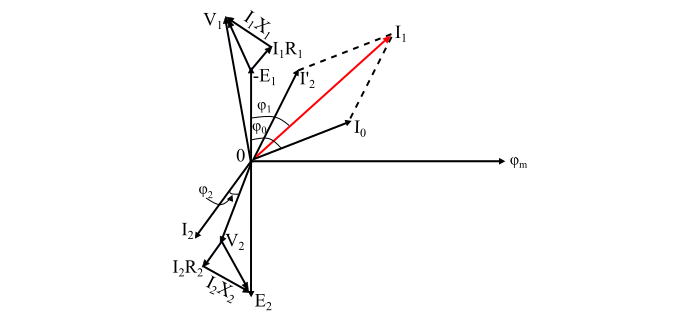When a load Impedance is connected across the secondary winding of the practical transformer, then the transformer is said to be loaded and draws a load which flows through the secondary winding and the load.

We shall consider following two cases for analysing the practical transformer −

## Case 1 – When the transformer is assumed to have no winding resistance and leakage flux

The figure shows a practical transformer with the assumption that the winding resistances and the leakage reactances are neglected. With this assumption,

$$\mathrm{𝑉_{1} = 𝐸_{1}\: and \:V_{2} = 𝐸_{2}}$$Consider an inductive load is connected across the secondary winding which causes the secondary current I2 to lag the secondary voltage V2 by an angle ϕ2. In this case, the total primary current I1 must fulfil two requirements −

• First, it must supply the no-load current I0 to produce the iron losses and the magnetic flux in core of the transformer.
• Secondly, it must supply a current I’2 to neutralise the demagnetising effect of secondary current I2.

The magnitude of current I’2 is given by,

$$\mathrm{𝑁_{1}I'_{2}= 𝑁_{2}I_{2}}$$

$$\mathrm{⇒ I'_{2}=\frac{𝑁_{2}}{𝑁_{1}}I_{2} = 𝐾I_{2}}$$

Therefore, the total primary current drawn by a practical transformer under loaded condition is given the phasor sum of I’2 and I0 i.e.

$$\mathrm{𝑰_{𝟏} = 𝑰'_{𝟐}+ 𝑰_{𝟎}}$$

Where,

$$\mathrm{𝐼’_{2} = − 𝐾I_{2}}$$

The negative sign shows that the current I’2 is 180° out of phase with current I2.The phasor diagram shows that both the emfs E1 and E2 lag behind the mutual flux (ϕm) by 90°. The current I’2 denotes the portion of primary current which neutralise the demagnetising effect of the secondary current (I2). Therefore, the current I’2 must be in anti-phase with I2. The phasor I0 represents the no-load current of the transformer. The phasor sum of I’2 and I0 represents the total primary current (I1). Hence,

$$\mathrm{primary\:power\: factor = cos \varphi_{1}}$$

$$\mathrm{Secondary\: power\: factor = cos \varphi_{2}}$$

Therefore,

$$\mathrm{Input\: power,𝑃_{1} = 𝑉_{1}𝐼_{1} cos \varphi_{1}}$$

$$\mathrm{Output \:power, 𝑃_{2} = V_{2}I_{2} cos \varphi_{2}}$$

## Case 2 – When the transformer has winding resistance and leakage flux

The figure shows a practical transformer having winding resistances and leakage reactances. This is the actual case that exits in a practical transformer. Here, some of the applied voltage is dropped in the primary winding resistance R1 and leakage reactance X1, thus the primary EMF (E 1) will be less than the applied voltage V1.

similarly, there being a voltage drop in the secondary winding resistance R2 and the leakage reactance X2 so that the voltage across the secondary winding terminals V2 will be less than the secondary EMF (E2).Now, consider an inductive load connected across the secondary winding of the transformer which causes the secondary current I2 to lag behind the secondary voltage V2 by an angle ϕ2 and the total primary current (I1) must fulfil two requirements −

• First, it must supply the no-load current I0 to produce the iron losses and the magnetic flux in core of the transformer.
• Secondly, it must supply a current I’2 to neutralise the demagnetising effect of secondary current I2.

The magnitude of current I'2 is given by,

$$\mathrm{𝑁_{1}I'_{2}= 𝑁_{2}I_{2}}$$

$$\mathrm{⇒ I'_{2} =\frac{𝑁_{2}}{𝑁_{1}}I_{2} = 𝐾I_{2}}$$

Therefore, the total primary current drawn by a practical transformer under loaded condition is given the phasor sum of I’2 and I0 i.e.

$$\mathrm{𝑰_{𝟏} = 𝑰'_{𝟐} + 𝑰_{𝟎}}$$

Where,

$$\mathrm{𝐼’_{2} = − 𝐾I_{2}}$$

The negative sign shows that the current I’2 is 180° out of phase with current I2.

Now, by applying KVL in primary loop and secondary loop, we get the applied primary voltage (V1) and secondary terminal voltage (V2) as,

$$\mathrm{𝑽_{𝟏} = −𝑬_{𝟏} + 𝑰_{𝟏}(𝑹_{𝟏} + 𝒋𝑿_{𝟏})}$$

$$\mathrm{⇒ 𝑽_{𝟏} = −𝑬_{𝟏} + 𝑰_𝟏𝒁_𝟏}$$

And,

$$\mathrm{𝑽_{𝟐} = 𝑬_{𝟐} − 𝑰_{𝟐}(𝑹_{𝟐} + 𝒋𝑿_{𝟐})}$$

$$\mathrm{⇒ 𝑽_{𝟐} = 𝑬_{𝟐} − 𝑰_𝟐𝒁_𝟐}$$

The bold letters shows the phasor sum.From the phasor diagram, it can be seen that both the EMFs E1 and E2 lag behind the mutual flux (ϕm) by 90°. The current I’2 represents the primary current to counteract the demagnetising effect of secondary current (I2) which in anti-phase with I2. The current I0 is the no-load current of the transformer. Thus, the total primary current (I1) is obtained by the phasor sum of I’2 and I0.

Also, the primary voltage (V1) is obtained by adding (phasor sum) the drop I1R1 and I1X1 to the counter EMF (– E1). The secondary terminal voltage V2 is obtained by subtracting (phasor difference) I2R2 and I2X2 from EMF (E2).

The input and output power factors are given by,

$$\mathrm{Input\:power \:factor\: = cos\varphi_1}$$

$$\mathrm{Output\: power\: factor\: = cos\varphi_2}$$

Also, the input and output powers of the transformer is given by,

$$\mathrm{Input\: power,\:𝑃1 = 𝑉_{1}𝐼_{1} cos \varphi_1}$$

$$\mathrm{Output \:power, \:𝑃2 = V_{2}I_{2} cos \varphi_2}$$

## Numerical Example

A 440/120 V single phase transformer takes no-load current of 6 A at 0.3 lagging power factor. If the secondary winding supplies a current of 100 A at a power factor 0.85 lagging. Determine the current taken by the primary winding.

## Solution

Here, the primary current I1 is given by phasor sum of I’2 and I0, thus,

$$\mathrm{cos\varphi_0 = 0.3;\:\: \therefore\:\: \varphi_0 = 72.54°}$$

$$\mathrm{cos\varphi_2 = 0.85;\:\: \therefore \:\:\varphi_2 = 31.79°}$$

Now, the transformation ratio is,

$$\mathrm{𝐾 =\frac{V_{2}}{𝑉_{1}}=\frac{120}{440} =\frac{3}{11}}$$

Therefore,

$$\mathrm{I'_{2}= 𝐾I_{2} = (\frac{3}{11}) × 100 = 27.27 A}$$Refer the phasor diagram, the angle between I’2 and I0 is

$$\mathrm{\theta = 72.54 − 31.79 = 40.75°}$$

Now, using the parallelogram law of vector addition, the primary current is

$$\mathrm{I_{1} = \sqrt{(I'_{2})^2 + (𝐼_{0})^2 + 2I_{0}I'_{2}cos \theta}}$$

$$\mathrm{⇒ 𝐼_{1} = \sqrt{(27.27)^2 + (6)^2 + (2 × 6 × 27.27 × cos 40.75)} = 32.05 A}$$